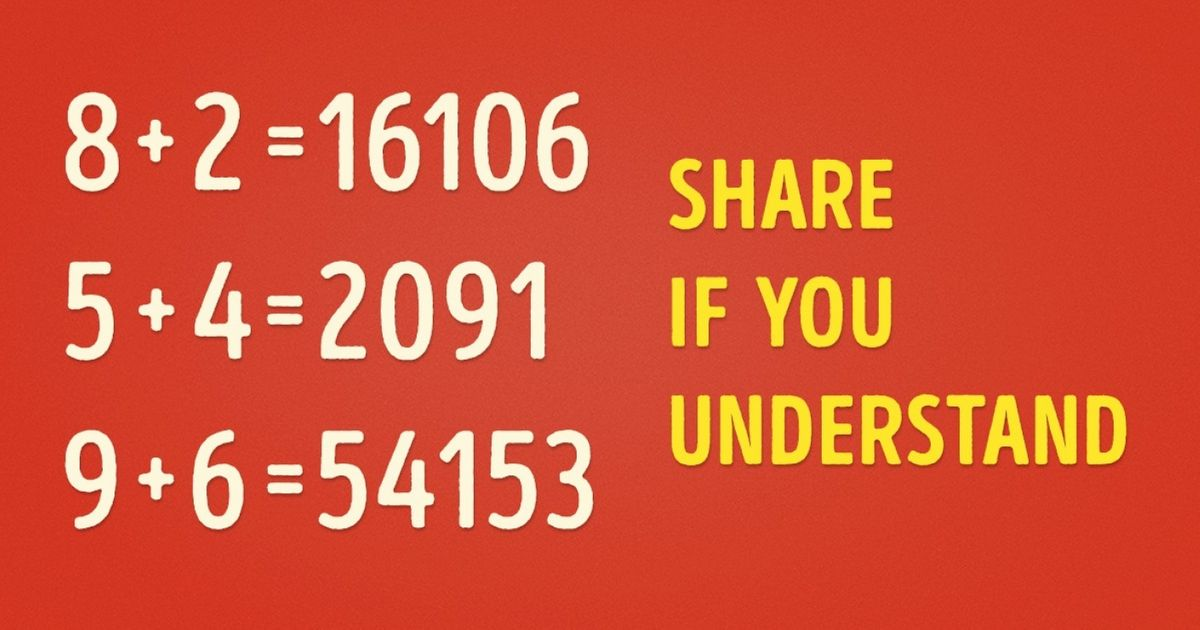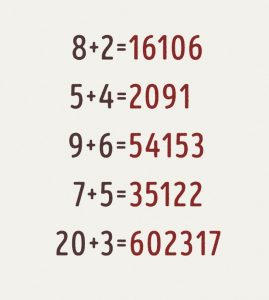# If You Can Solve This Math Equation, Then You Really Are A Genius

Because of its rigorous and complicated rules, mathematics is disliked by many students. However, this conundrum defies all known mathematical principles. Are you able to figure it out?

Try to figure out what these equations have in common:Avoid focusing on the big number in the “answer.” It’s better to conceive of it as a collection of smaller numbers rather than a single large number.
Every number following the equals sign reflects the outcomes of three sums generated by multiplying, adding, and subtracting the two numbers before the equals sign.

For example, 8 + 2 = 16106

8 x 2 = 16
8 + 2 = 10
8 – 2 = 6

The same concept applies to the remaining equations. Do you believe your buddies will be able to get it as quickly as you did now that you know?